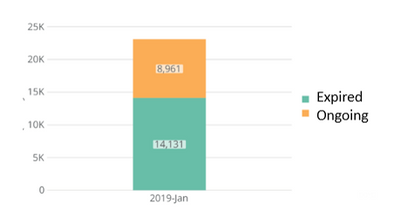# how to use the percentage of a category as the summary number

Hello, I have this card that looks like this (Showing only one month's data)What I want to show in the summary number is the percentage of expired category(14,131 / 8,961+14,131) that is made available when you hover over the chart (61.19%). How do I do that for the summary number? Thanks!

• You would need to create a Beast Mode basically says:

`SUM(CASE WHEN `Category` like 'Expired' THEN `Value` END)/SUM(`Value`)`

Then select that Beast Mode as your summary number. (one tip on summary numbers, they will only allow you to use a beast mode if the beast mode is an aggregation. Can't put something like 1+1 would instead need SUM(1+1))

Best of luck,
Valiant

`SUM(CASE WHEN `Category` like 'Expired' AND MONTH(`Date Field`)=MONTH(CURDATE()) THEN `Value` END)/SUM(CASE WHEN MONTH(`Date Field`)=MONTH(CURDATE()) THEN `Value` END)`